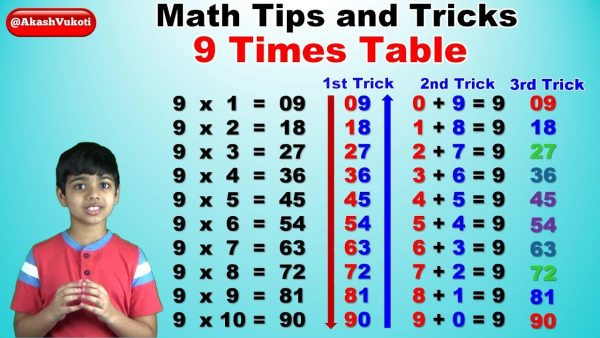News

# 5 Tips to Memorize Multiplication TablesWritten by
You Are Here » Home » News» 5 Tips to Memorize Multiplication TablesMemorizing and understanding multiplication tables is an important skill that kids need to master from an early stage. It is a basic building block to create the core foundation for various math concepts like fractions, percentage, factorization, and many more. Memorizing the multiplication tables is helpful to gain confidence while doing simple calculations in daily life. Learning multiplication tables requires dealing with abstract numbers, which may seem a little daunting for many students. A multiplication chart is a helpful resource to memorize tables for students.

A multiplication chart is a tabular representation of times tables that show the products of two numbers. Usually, one set of numbers is written on the left column, and another set is written as the top row with products of these numbers listed as well. It is a super handy tool to learn multiplication tables. There are various other activities and engaging exercises that train a child’s brain without actively memorizing multiplication tables.

These activities help children familiarize themselves with multiplication tables and feel comfortable using them without the pressure of knowing them by heart. Unfortunately, some children still struggle to learn multiplication tables slightly more than others, even with all these activities. Here are some additional tricks or tips for memorizing multiplication tables from 1 to 20.

## Tips for Learning Multiplication Tables Fast

### Familiarize Children with Multiplication Tables

An important thing to do while introducing multiplication tables is to make kids familiar with the properties and use of multiplication tables. It will help kids to memorize them better. There are various ways to help children familiarize with multiplication tables like table charts, flashcards, writing tables, reciting multiplication tables, etc.

### Use fingers to Memorize the Easy Numbers

Easy numbers are those which follow the most obvious and visible pattern. These numbers are an enjoyable way to kick-start while memorizing the multiplication tables from 1 to 20. For example, here are some easy ways to begin with multiplication tables:

• Multiplication with the number 0 is zero.
• Multiplication with the number 1 is the same value as before the multiplication.
• Multiplication with the number 2 is the same as doubling the number, For example, 3×2 = 6, 3 + 3 = 6,
• Multiplication with the number 5 follows the product ends up in 5 or 0.
• Multiplication with the number 10 is simply adding 0 to the end of the number. For example, 3 x 10 = 30, 4 x 10 = 40, 5×10 = 50, etc.

### Utilize Online Learning

Once the child establishes a basic understanding of tables, you can search online for worksheets and games to see how much your child has learned. Using games and interactive worksheets can help in grabbing a child’s attention to perform better. They won’t feel the stress or fear of assessment, which will help them learn fast in a fun-filled environment.

### Learn Tricks

Math is full of tricks to learn various concepts. Learning multiplication tables also becomes comfortable with these tricks. You can easily find some of them on the internet. For example, A trick to remember the multiplication table of 9 is to keep the second digit one lesser than its predecessor-: 9, 18, 27, 36, 45, 54, 63, 72, 81, 90.

### Practice Skip Counting

Skip-counting is the best math skill for memorizing multiplication tables. An amazing fact about multiplication tables is that they have a pattern that children can quickly learn through skip-counting. By using skip counting, a child will not only learn to memorize the multiplication table but will also have fun while doing it. For example, skip-counting by three would be 3, 6, 9, 12, etc.

### Conclusion:

Multiplication tables are not as hard to memorize as some people think. All it needs is finding the right tools and techniques. While introducing students to multiplication tables for the first time, you should first start by telling them “why” by explaining multiplication. After this, follow the steps and the activities you find most interesting. You can find some of these activities at Cuemath.com.

HitzGh is committed to complying with the requirements of the Digital Millennium Copyright Act (DMCA) and other applicable intellectual property laws. Therefore, we will promptly respond to any and all take-down requests that meet the necessary legal criteria. If you are a copyright owner and believe that a file uploaded to hitxgh.com infringes upon your rights, please contact us at [email protected] to submit a request. Thank you for your cooperation.